Search datasheet (1.687.043 components) Search fieldPart namePart descriptionAbout site Manufacturers list ChipFindIC search engine AllXrefCross-reference database### Datasheet: L200CV (STMicroelectronics)

ADJUSTABLE VOLTAGE and CURRENT REGULATOR
Download:PDFZIP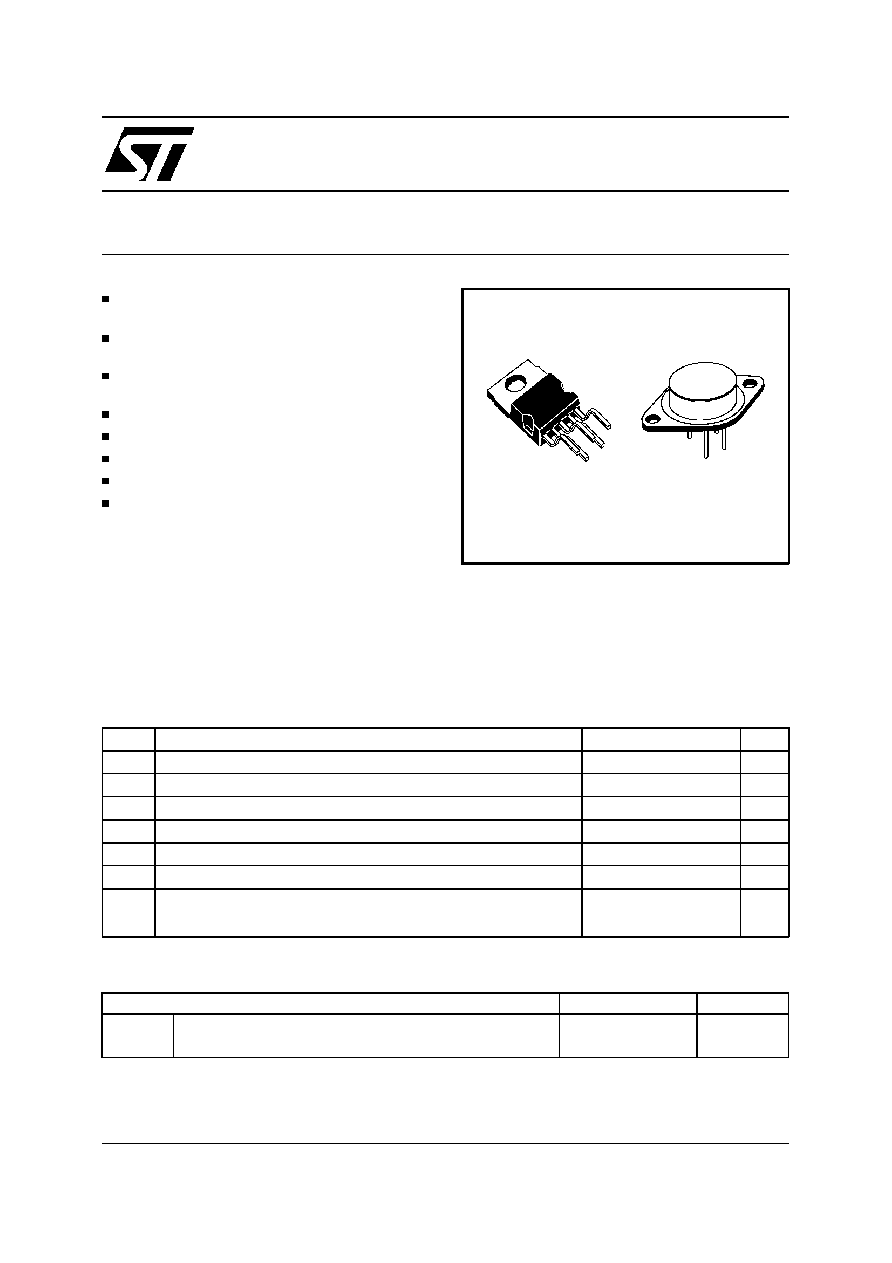L200
ADJUSTABLE VOLTAGE AND CURRENT REGULATOR
ADJUSTABLE OUTPUT CURRENT UP TO 2 A
(GUARANTEED UP TO T
j
= 150
C)
ADJUSTABLE OUTPUT VOLTAGE DOWN TO
2.85 V
INPUT OVERVOLTAGE PROTECTION (UP TO
60 V, 10 ms)
SHORT CIRCUIT PROTECTION
OUTPUT TRANSISTOR S.O.A. PROTECTION
THERMAL OVERLOAD PROTECTION
LOW BIAS CURRENT ON REGULATION PIN
LOW STANDBY CURRENT DRAIN
DESCRIPTION
The L200 is a monolithic integrated circuit for volt-
age and current programmable regulation. It is
available in Pentawatt
package or 4-lead TO-3
metal case.Current limiting, power limiting, thermal
shutdown and input overvoltage protection (up to
January 2000
Pentawatt
TO-3 (4 lead)
60 V) make the L200 virtually blow-out proof.
The L200 can be used to replace fixed voltage
regulators when high output voltage precision is
required and eliminates the need to stock a range
of fixed voltage regulators.
Symbol
Parameter
Value
Unit
V
i
DC Input Voltage
40
V
V
i
Peak Input Voltage (10 ms)
60
V
V
i-o
Dropout Voltage
32
V
I
o
Output Current
internally limited
P
tot
Power Dissipation
internally limited
T
stg
Storage Temperature
-55 to 150
C
T
op
Operating Junction Temperature for L200C
-25 to 150
C
for L200
-55 to 150
C
ABSOLUTE MAXIMUM RATINGS
TO-3
Pentawatt
R
th j-case
Thermal Resistance Junction-case
Max
4
C/W
3
C/W
R
th j-amb
Thermal Resistance Junction-ambient
Max
35
C/W
50
C/W
THERMAL DATA
1/12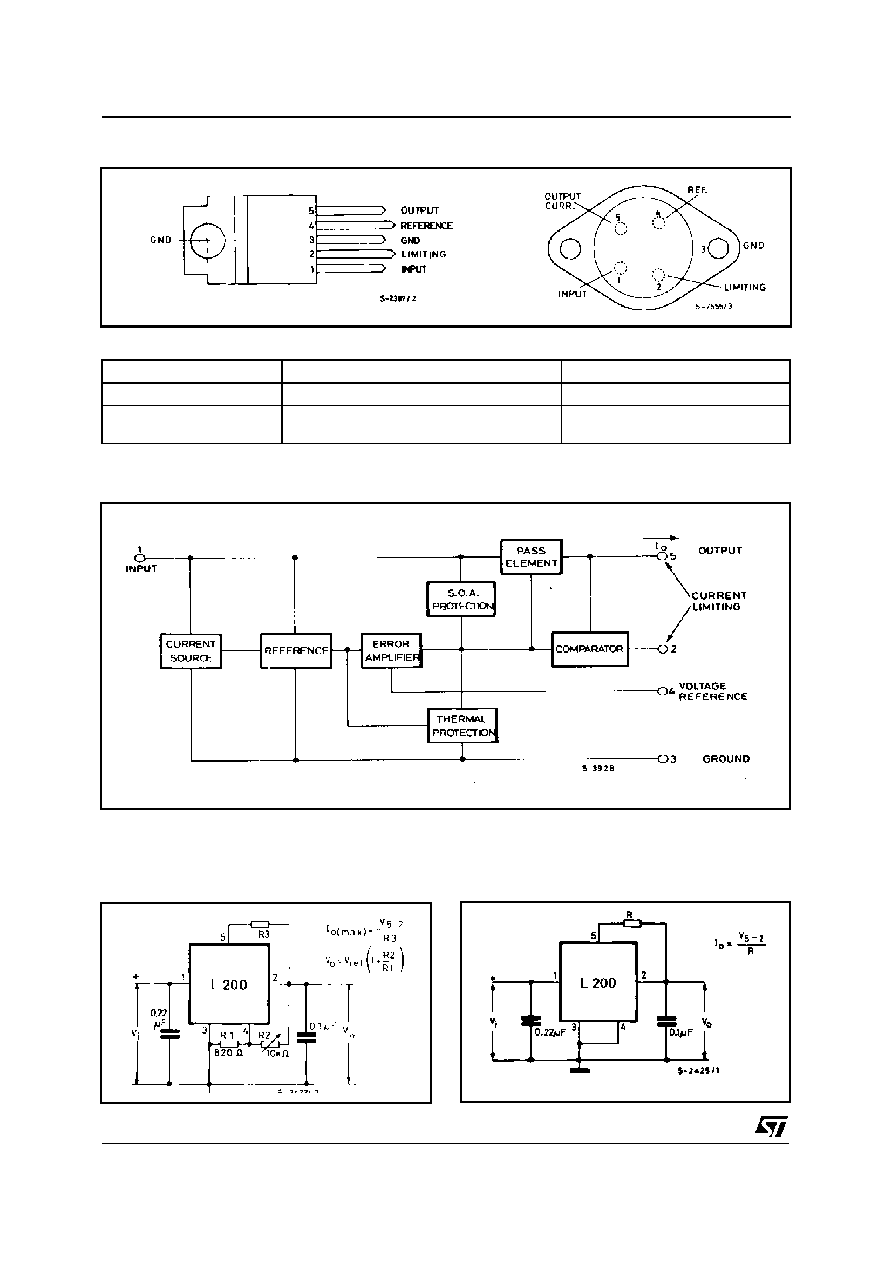2/12
CONNECTION DIAGRAMS AND ORDER CODES (top views)
BLOCK DIAGRAM
APPLICATION CIRCUITS
Figure 1. Programmable Voltage Regulator
with Current Limiting
Figure 2. Programmable Current Regulator.
Type
Pentawatt
TO-3
L200
L200 T
L200 C
L200 CH
L200 CV
L200 CT
L200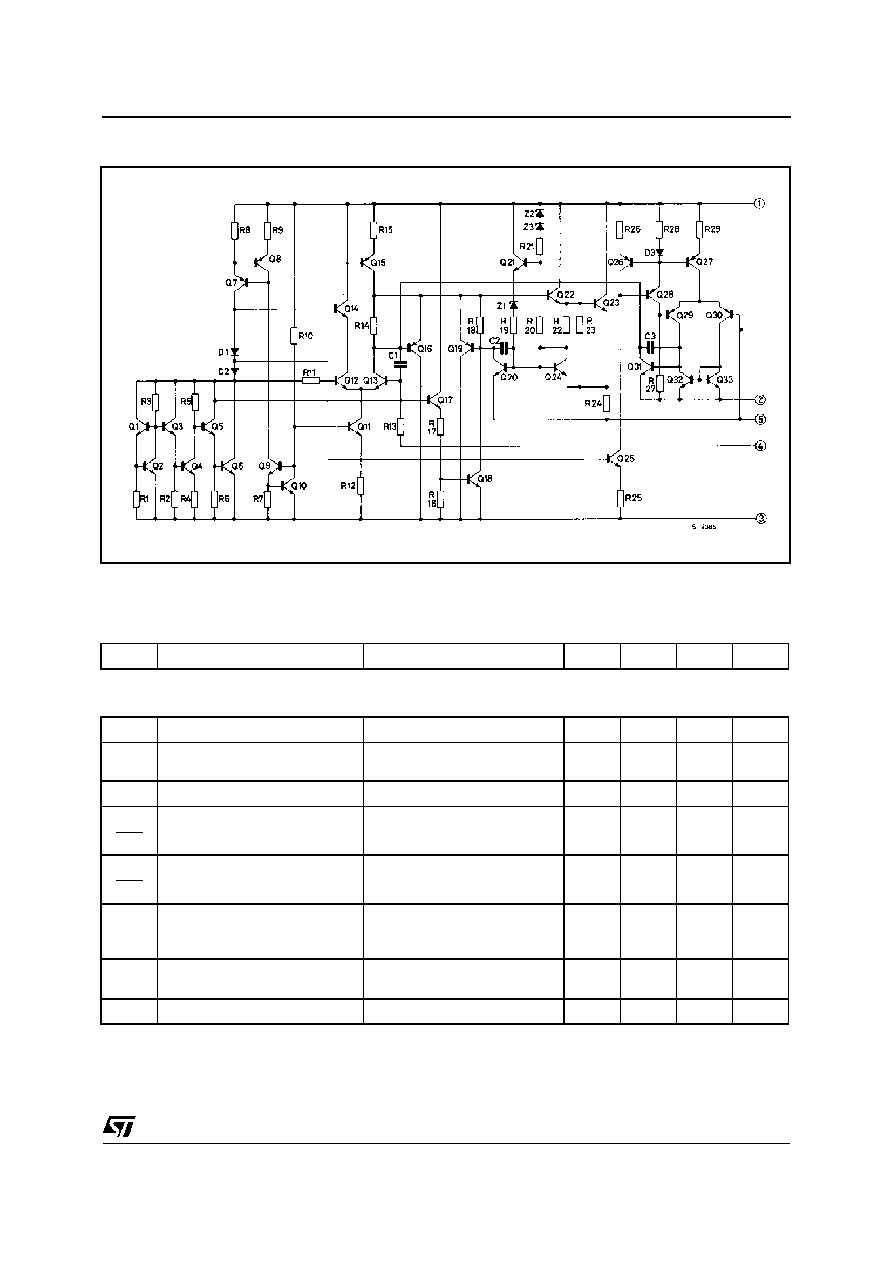SCHEMATIC DIAGRAM
Symbol
Parameter
Test Conditions
Min.
Typ.
Max.
Unit
VOLTAGE REGULATION LOOP
I
d
Quiescent drain Current (pin 3)
V
i
= 20 V
4.2
9.2
mA
e
N
Output Noise Voltage
Vo = Vref
I
o
= 10 mA
B = 1 MHz
80
V
V
o
Output Voltage Range
I
o
= 10 mA
2.85
36
V
V
o
V
o
Voltage Load Regulation
(note 1)
I
o
= 2 A
I
o
= 1.5 A
0.15
0.1
1
0.9
%
%
V
i
V
o
Line Regulation
V
0
= 5 V
V
i
= 8 to 18 V
48
60
dB
SVR
Supply Voltage Rejection
V
0
= 5 V
I
o
= 500 mA
V
i
= 10 V
pp
f = 100 Hz (note 2)
48
60
dB
V
i-o
Droupout Voltage between Pins 1
and 5
I
o
= 1.5 A
V
0
2%
2
2.5
V
V
ref
Reference Voltage (pin 4)
V
i
= 20 V
I
o
= 10 mA
2.64
2.77
2.86
V
ELECTRICAL CHARACTERISTICS (T
amb
= 25
C, unless otherwise specified)
3/12
L200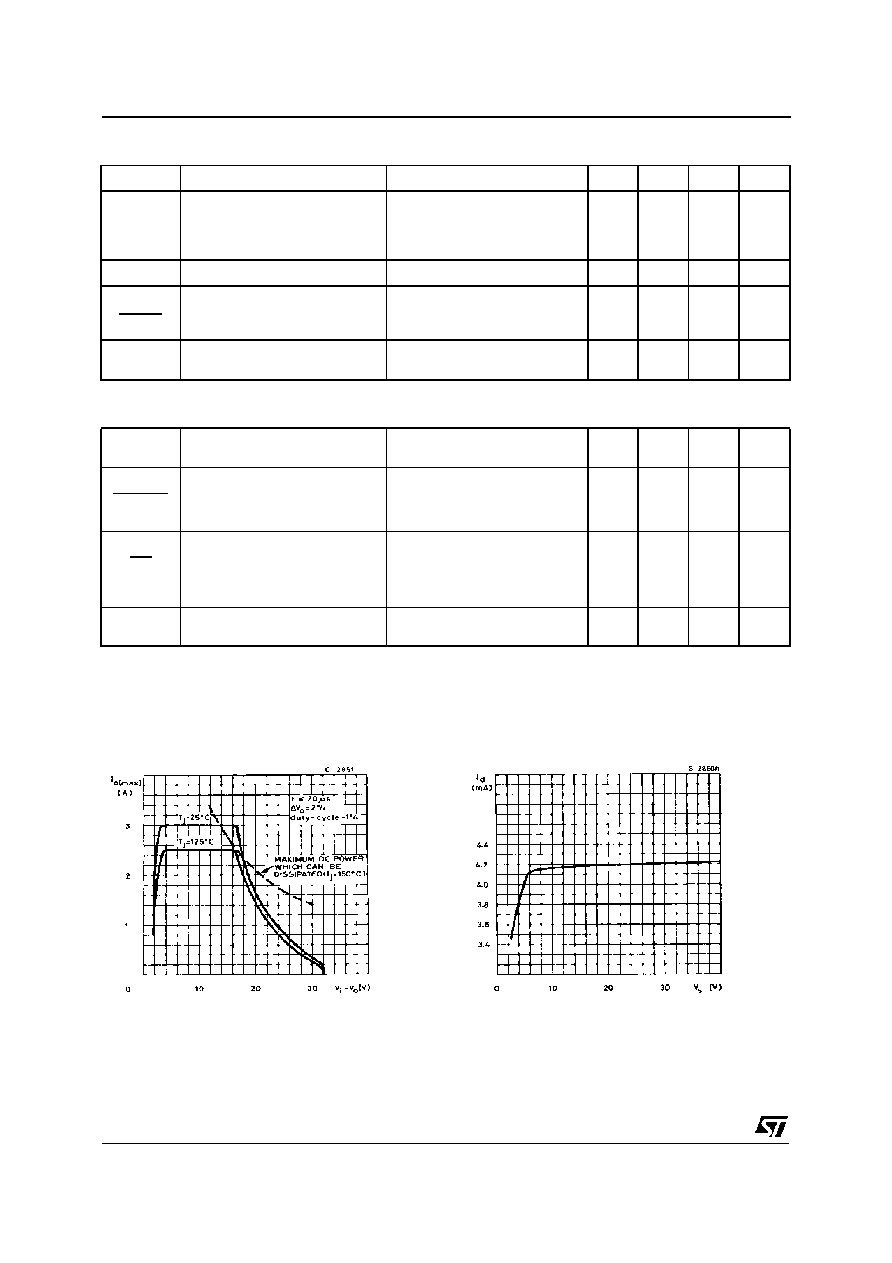Symbol
Parameter
Test Conditions
Min.
Typ.
Max.
Unit
V
ref
Average Temperature Coefficient
of Reference Voltage
V
i
= 20 V
Io = 10mA
for Tj = - 25 to 125
C
for Tj = 125 to 150
C
-0.25
-1.5
mV/
C
mV/
C
I
4
Bias Current and Pin 4
3
10
A
I
4
T
I
4
Average Temperature
Coefficient (pin 4)
-0.5
%/�
C
Z
o
Output Impedance
V
i
= 10 V
V
o
= V
ref
I
o
= 0.5 A
f = 100 Hz
1.5
m
CURRENT REGULATION LOOP
V
SC
Current Limit Sense Voltage
between Pins 5 and 2
V
i
= 10 V
V
o
= V
ref
I
5
= 100 mA
0.38
0.45
0.52
V
V
SC
T
V
SC
Average Temperature
Coefficient of V
SC
0.03
%/�
C
I
o
I
o
Current Load Regulation
Vi = 10 V
Vo = 3V
I
o
= 0.5 A
I
o
= 1A
I
o
= 1.5 A
1.4
1
0.9
%
%
%
I
SC
Peak Short Circuit Current
V
i
- V
0
= 14 V
(pins 2 and 5 short circuited)
3.6
A
ELECTRICAL CHARACTERISTICS (continued)
4/12
Figure 3. Typical Safe Operating Area
Protection.
Figure 4. Quiescent Current vs. Supply
Voltage.
Note 1: A load step of 2 A can be applied provited that input-output differential voltage is lower than 20 V (see Figure 3).
Note 2: The same performance can be maintained at higher output levels if a bypassing capacitor is provited between pins 2 and 4.
L200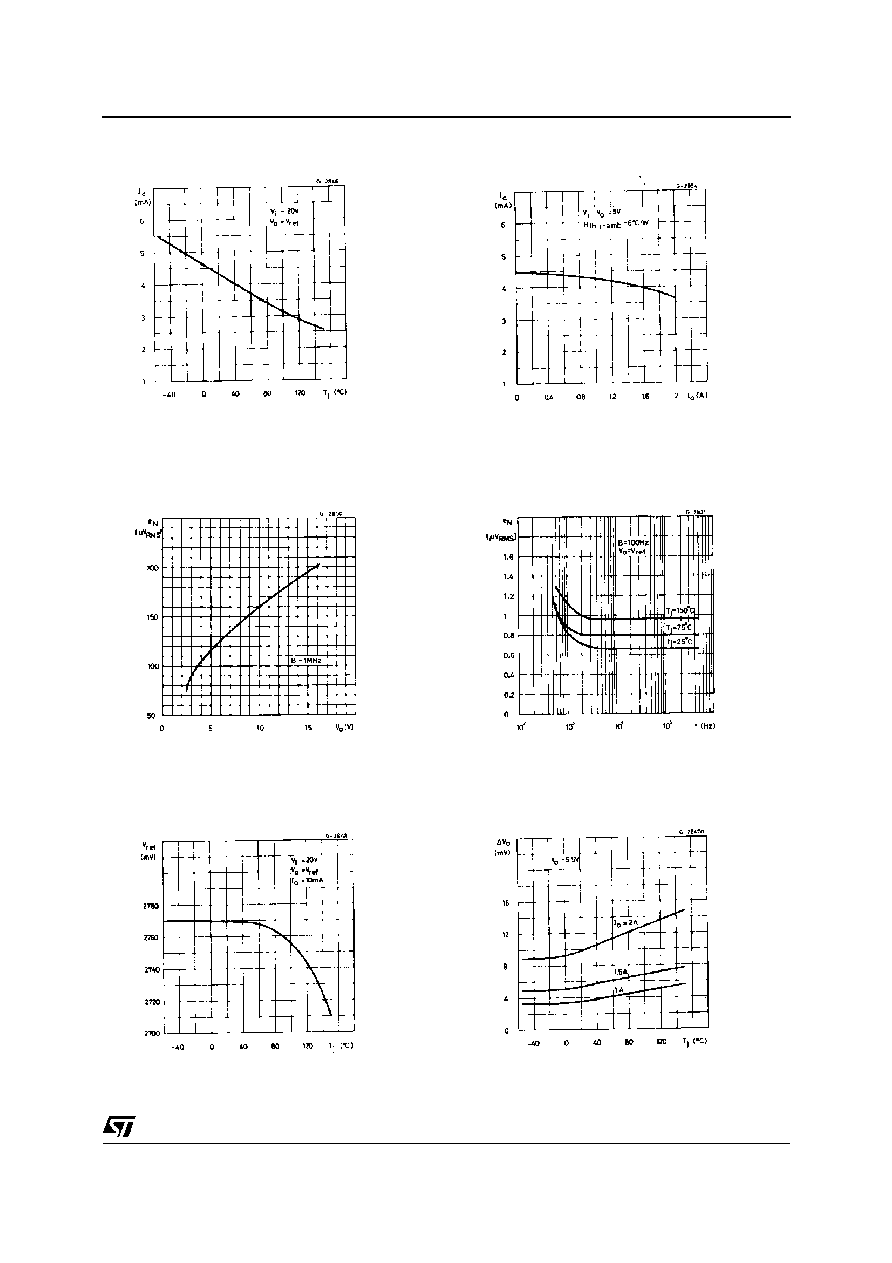Figure 5. Quiescent Current vs. Junction
Voltage.
Figure 6. Quiescent Current vs. Output
Current.
Figure 7. Output Noise Voltage vs. Output
Voltage.
Figure 8. Output Noise Voltage vs.
Frequency.
Figure 9. Reference Voltage vs. Junction
Temperature.
Figure 10. Voltage Load Regulation vs.
Junction Temperature.
5/12
L200© 2019 • ICSheet• Contact form• Main page